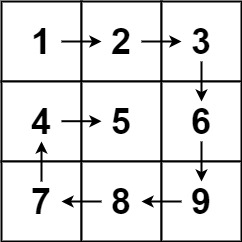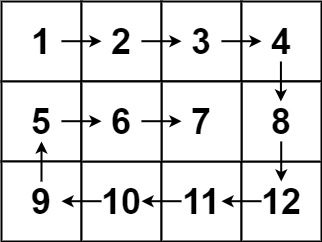# LeetCode Notes: Spiral Matrix

## Question

Given an `m x n` `matrix`, return all elements of the `matrix` in spiral order.

Example 1:Input: matrix = [[1,2,3],[4,5,6],[7,8,9]]

Output: [1,2,3,6,9,8,7,4,5]

Example 2:Input: matrix = [[1,2,3,4],[5,6,7,8],[9,10,11,12]]

Output: [1,2,3,4,8,12,11,10,9,5,6,7]

Constraints:

• `m == matrix.length`
• `n == matrix[i].length`
• `1 <= m, n <= 10`
• `-100 <= matrix[i][j] <= 100`

## Solution

Analysis:

1. Set `up`/ `down`/ `left`/ `right` start and end position variables
2. Traverse horizontally and vertically according to the requirements, each time one direction is traversed, the start and end positions will be reduced to achieve the spiral effect

Code:

``````/**
* @param {number[][]} matrix
* @return {number[]}
*/
var spiralOrder = function(matrix) {
// is empty judgment
if(matrix.length === 0) return []

// Store the result array
const result = []

// Get the initial element position information
let left = 0, right = matrix.length-1,
top = 0, bottom = matrix.length-1

while(true){
// Traverse to the right
for(let i = left; i <= right; i++){
result.push(matrix[top][i])
}
// The traversal is over, the top value just traversed is no longer used, the upper boundary needs to move down by +1, if the top value exceeds the bottom value, it means that the vertical traversal has ended, exit the loop
if(++top> bottom) break

// traverse down
for(let i = top; i <= bottom; i++){
result.push(matrix[i][right])
}
// The traversal is over, the right value just traversed is no longer used, the right boundary needs to be moved to the left by -1, if the right value is less than the left value, it means that the horizontal traversal has ended, exit the loop
if(--right <left) break

// Traverse left
for(let i = right; i >= left; i--){
result.push(matrix[bottom][i])
}
// The traversal is over, the bottom value just traversed is no longer used, the lower boundary needs to move up by -1, if the bottom value is less than the top value, it means that the vertical traversal has ended, exit the loop
if(--bottom <top) break

// traverse upward
for(let i = bottom; i >= top; i--){
result.push(matrix[i][left])
}
// The traversal is over, the left value just traversed is no longer used, the left boundary needs to move to the right by +1, if the left value is greater than the right value, it means that the horizontal traversal has ended, exit the loop
if(++left> right) break

}

return result

};
``````